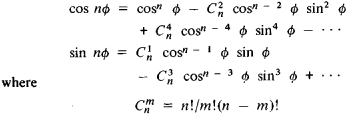# De Moivre's Theorem

(redirected from De Moivre theorem)

## De Moivre's theorem

[də′mwäv·rəz ‚thir·əm]
(mathematics)
The n th power of the quantity cos θ + i sin θ is cos n θ + i sin n θ for any integer n.

## De Moivre’s Theorem

a rule for raising a complex number to the nth power when the number is expressed in polar form as

z; = p(cos φ + i sin φ)

According to the rule, the modulus ρ of the complex number is raised to the given power and the amplitude φ is multiplied by the exponent:

zn = [p(cos φ + i sin φ)]n

= pn(cos nφ + i sin nφ) The theorem was devised by A. De Moivre in 1707, and its modern form was proposed by L. Euler in 1748.

De Moivre’s theorem can easily be used to express cos nϕ and sin nϕ in terms of powers of cos φ and sin φ. If we set ρ = 1 in De Moivre’s theorem and separately equate the real and imaginary parts, then we getare binomial coefficients. Inversion of De Moivre’s theorem leads to a formula for extracting the root of a complex number.

Site: Follow: Share:
Open / Close